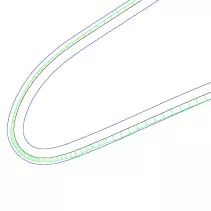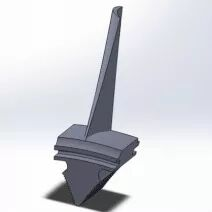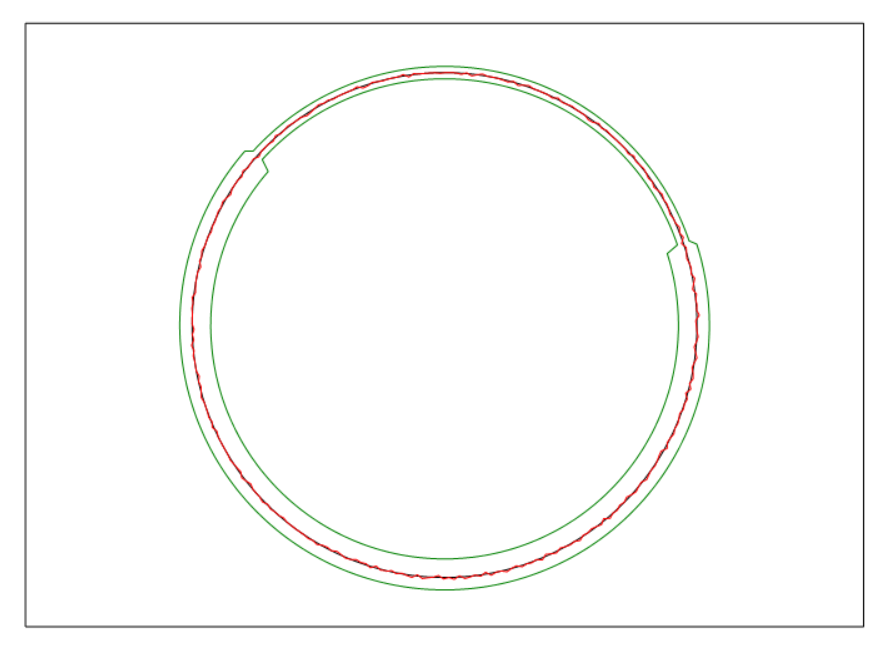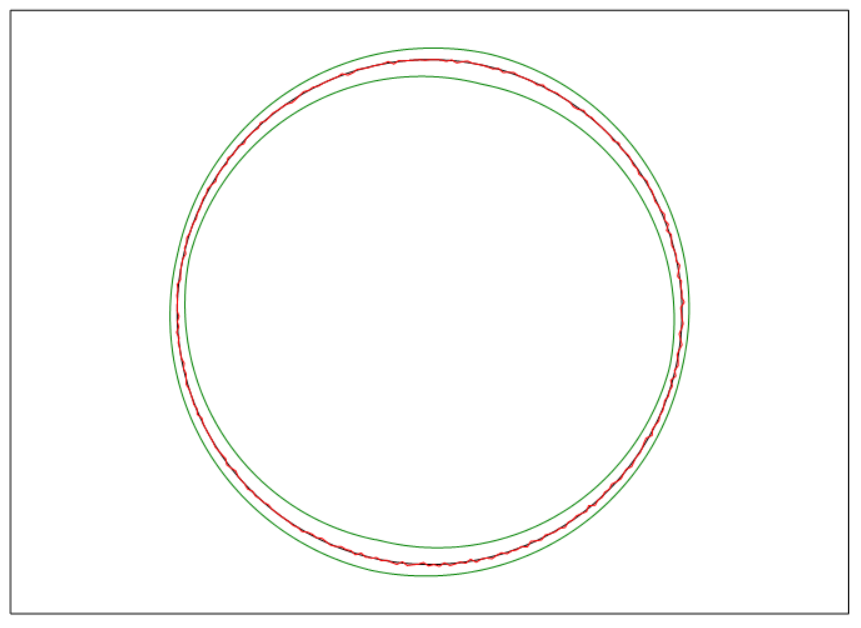# 变公差带评价方法1叶片机加工时余量图叶片图（图片来自网络）

QUINDOS软件中的“GenUprLwrTolValues”程序有三种生成公差带的方式，分别是生成定公差带；通过指定元素来产生公差带和按照点的顺序输入数值得到连续渐变的公差带。公差的数值被保存在目标元素NPTsA（上公差）和B（下公差）中。

2

2.1 分段不对称公差带

GenUprLwrTolValues(DST=SECT,MMD=Y,

PIV=(1..100,101..102,103..200,201..202,203..301),

LIV=(-0.03..-0.03,-0.03..-0.01,-0.01..-0.01,-0.01..-0.03,-0.03..-0.03),

UIV=(0.02..0.02,0.02..0.01,0.01..0.01,0.01..0.02,0.02..0.02)DO (NAM=N, BGN=1, END=301)
GETVALS  (OBJ=SECT.NOM.PTS(N), TYP=ELE, RDS=A, REA=U)
PUTVALS  (OBJ=SECT.ACT.PTS(N), TYP=ELE, RDS=A, VAL=U)
ENDDO
CPYOBJ  (FRM=SECT3, TO =UPP_TOL, TYP=ELE, SRC=LDB, DST=LDB)DO (NAM=N, BGN=1, END=301)
GETVALS  (OBJ=SECT.NOM.PTS(N), TYP=ELE, RDS=B, REA=U)
PUTVALS  (OBJ=SECT.ACT.PTS(N), TYP=ELE, RDS=A, VAL=U)
ENDDO
CPYOBJ  (FRM=SECT, TO =LOW_TOL, TYP=ELE, SRC=LDB, DST=LDB)PLS_DELPAGE  (ALL=Y)
DRWPLY  (NAM=LOW_TOL, ASC=Y, DEV=Y, FAC=20.0, DRP=0, PEN=3, A_O=XY)
DRWPLY  (NAM=UPP_TOL, ASC=Y, DEV=Y, FAC=20.0, DRP=0, PEN=3, A_O=XY)
DRWPLY  (NAM=SECT, ASC=Y, DEV=Y, FAC=1.0, DRP=0, PEN=1, A_O=XY)
DRWPLY  (NAM=SECT_RCO, ASC=Y, DEV=Y, FAC=20.0, PEN=2, A_O=XY)3

连续渐变不对称公差带

GenUprLwrTolValues  (DST=SECT3, MMD=Y,
PIV=(1..75,76..150,151..225,226..301),
LIV=(-0.03..-0.01,-0.01..-0.03,-0.03..-0.01,-0.01..-0.03),
UIV=(0.02..0.01,0.01..0.02,0.02..0.01,0.01..0.02)## 公差修饰符号CF，CT，CZ的理解和应用#### 暂无评论• 180
文章
• 0
评论
• 0
粉丝
• 0
点赞
##### 最近文章
• 海克斯康大学最新公...
海克斯康大学— 2019年全新课程安排 —贯穿制造全流程，总有一门你需要的铸造大国工匠，成就智能制造。依托海克斯康制造智能全...